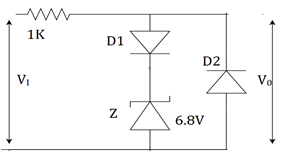Courses

# Test: Voltage Regulation Using Zener Diode

## 10 Questions MCQ Test Electronic Devices | Test: Voltage Regulation Using Zener Diode

Description
This mock test of Test: Voltage Regulation Using Zener Diode for Electrical Engineering (EE) helps you for every Electrical Engineering (EE) entrance exam. This contains 10 Multiple Choice Questions for Electrical Engineering (EE) Test: Voltage Regulation Using Zener Diode (mcq) to study with solutions a complete question bank. The solved questions answers in this Test: Voltage Regulation Using Zener Diode quiz give you a good mix of easy questions and tough questions. Electrical Engineering (EE) students definitely take this Test: Voltage Regulation Using Zener Diode exercise for a better result in the exam. You can find other Test: Voltage Regulation Using Zener Diode extra questions, long questions & short questions for Electrical Engineering (EE) on EduRev as well by searching above.
QUESTION: 1

### The percentage voltage regulation (VL) is given by_________

Solution:

The change in the output voltage from no load to full load condition is called as voltage regulation, where VNL is the voltage at no load condition. It is used to maintain a nearly constant output voltage. If the regulation is high, the output voltage is stable.

QUESTION: 2

### The limiting value of the current resistor used in a Zener diode (when used as a regulator)

Solution:

When the input voltage is maximum, the load current is minimum, the Zener current should not increase the maximum rated value. Therefore there should be a minimum value of resistor.

QUESTION: 3

### When the regulation by a Zener diode is with a varying input voltage, what happens to the voltage drop across the resistance?

Solution:

When the input voltage varies, the input current also varies. This makes more current to flow in the diode. This increase in the current should balance a change in the load current. Hence the voltage drop increases across the resistor.

QUESTION: 4

In the given limiter circuit, an input voltage Vi=10sin100πt is applied. Assume that the diode drop is 0.7V when it’s forward biased. The zener breakdown voltage is 6.8V.The maximum and minimum values of outputs voltage are _______Solution:

With VI= 10V when maximum, D1 is forward biased, D2 is reverse biased. Zener is in breakdown region. VOMAX=sum of breakdown voltage and diode drop=6.8+0.7=7.5V. VOMIN=negative of voltage drop=-0.7V. There will be no breakdown voltage here.

QUESTION: 5

Determine the maximum and minimum values of load current for which the Zener diode shunt regulator will maintain regulation when VIN=24V and R=500Ω. The Zener diode has a VZ=12V and (IZ)MAX=90mA.

Solution:

The current through the resistance R is given by, I=(VIN-VZ)/R= (24-12)/500=24mA. (IL)MAX=I-(IZ)MIN=24-3=21mA .This current is less than (IZ)MAX. So, we assume that all the input current flows through the Zener diode. Under this condition, (IL)MINis 0mA.

QUESTION: 6

Determine the minimum value of load resistance that can be used in the circuit with (IZ)Min=3mA. The input voltage is 10V and the resistance R is 500Ω. The Zener diode has a VZ=6V 0and (IZ)MAX=90mA.

Solution:

The I=(VIN-VZ)/R=(10-6)/500=8mA. (IL)MAX=I-(IZ)MIN=8-3=5mA. (RL)MIN=VZ/(IL)MAX=6/5m=1.2KΩ.

QUESTION: 7

A Zener regulator has to handle supply voltage variation from 19.5V to 22.5V. Find the magnitude of regulating resistance, if the load resistance is 6KΩ. The Zener diode has the following specifications: breakdown voltage =18V, (IZ)Min=2µA, maximum power dissipation=60mW and Zener resistance =20Ω.

Solution:

(PZ)MAX/rZ=(IZ)MAX2 . So, (IZ)MAX =60m/20=54.8µA. IL=VO/RL=18/6000=3mA.
RMAX=(VMin-VZ)/[( IZ)Min+( IL)MAX]=(19.5-18)/(2µ+3m)=500Ω.
RMin=(VMAX-VZ)/[( IZ)MAX+( IL)Min]=(22.5-18)/(54.8m+3m)=77.8Ω.

QUESTION: 8

A transistor series regulator has the following specifications: VIN=15V, VZ=8.3V, β=100, R=1.8KΩ, RL=2KΩ. What will be the Zener current in the regulator circuit?

Solution:

We know, VO=VZ-VBE=8.3-0.7=7.6V. VCE=VIN-V0=15-7.6=7.4V. So, IR=(VIN-VZ)/R=(15-8.3)/1.8m=3.72mA. IL=VO/RL=7.6/2000=3.8mA. IB=IL/ β=3.8mA/100=0.038mA. Finally, IZ=IR-IB=3.72-0.038=3.682mA.

QUESTION: 9

When is a regulator used?

Solution:

The regulator has following limitations: 1.It has low efficiency for heavy load currents 2. The output voltage changes slightly due to Zener impedance. Hence, it is used when there are small variations in load current and input voltage.

QUESTION: 10

A transistor in a series voltage regulator acts like a variable resistor. The value of its resistance is determined by _______

Solution:

The principle is based on the fact that a large fraction of the increase in input voltage appears across the transistor so that the output voltage remains to be constant. When input voltage is increased, the output voltage also increases which biases the transistor towards less current.